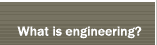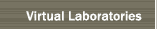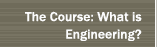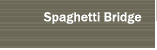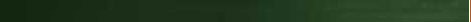### Virtual Laboratories - Experiments

view Virtual Laboratory Introduction >>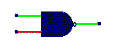Logic circuits. Binary logic elements are the basic building blocks for all digital devices. This project consists of creating logical circuits using elementary logic "gates" to effect computer calculations and robotic control. (Contributors: wak, mjk.)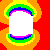Diffusion processes.What do the spreading of a toxic chemical, the cooling of a hot rock, and the absorption of medicine through the skin have in common? They are all the results of diffusion--a process which moves chemicals or heat from regions of high concentration to regions of low concentration. Explore these phenomena using Diffusion Simulator-- a tool for modeling and analyzing diffusion processes. (Contributor: mjk.)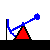Drilling for oil. One of the problems of petroleum engineering is determining the topology of an underlying oil-bearing stratum. In this exercise, the goal is to find this stratum by drilling a sequence of pilot holes and deducing its contour. (Contributors: ckg, wak, mjk.)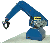Robotic arm control. This is a project involving the control of a two-segment robotic arm. The objective is to program the rotational motions of the segments so that the tip of the robotic arm traverses along a specified path. (Contributor: mjk.)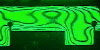Heat transfer in a duct. Deduce the rate at which heat is transfered from a heated, ribbed surface in an air duct. The project centers on obtaining measurements from interference holograms obtained during the running of an actual experiment. (Contributors: mjk, ch.)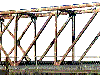Bridge designer. Design a truss bridge. Specify loadings and support nodes, and obtain the tension and compression forces on each of its members. (Useful for many statics problems, as well.) (Contributor: mjk.)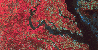How many trees? To obtain the timber value of a large remote area, estimate the number of trees from a Landsat image--a problem in statistical sampling. (Contributor: mjk.)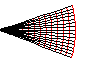Sound propagation. Sound propagation is affected by wind, temperature, and surfaces. Evaluate these effects by following the trajectories of acoustic rays through an adjustable propagation medium. (Contributors: mjk,phbb.)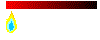Heat conduction. When heat is applied at one end of a metal bar, it is distributed by thermal conduction along the length of the bar. If the bar is not uniformly conductive, as in a bar with non-constant cross-sectional area, then that distribution of heat is affected. Can you deduce the cross-section of a bar from temperature profiles or probes? (Contributors: mhl,mjk.)[NOTE: This simulation uses JAVA frames whose implementations are not uniform across all Web browsers. Consequently, there will be minor operational differences and visual differences between earlier and later versions of MSIE and Netscape.]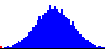Probability distributions. Probabilistic events occur throughout nature and science: measurement errors, molecular diffusion, games of chance-- all involve probabilistic events. How outcomes are affected by such events are estimated through the mathematics of statistics and probability. In this simulation, create mathematical expressions which contain random elements and observe the distribution of outcomes. (Contributors: jww,mjk.)

More to come. . .